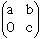Name: Tamaswati Who is asking: Student Question: How do I prove the assertion that "the determinant of an upper triangular matrix is the product of the diagonal entries" by mathematical induction? (Before I check this assertion for a few values of n how do I rephrase the assertion slightly so that n appears explicitly in the assertion?) Hi Tamaswati, What you want for n in this case is the "size" of the matrix. A matrix is a square array of numbers, say with n rows and n columns. We usually say an nxn matrix. You can start the induction at n = 1. A 1x1 matrix has only one entry, call it a. The determinant has value a and this is also the product of the diaginal entries. If it bothers you to think of a 1x1 matrix ten start the induction at n = 2. An upper triangular 2x2 matrix is of the formIts dertrminant is axc - 0xb = axc and the product of its diagonal entries is also axc. Now try the inductive step. Penny Go to Math Central